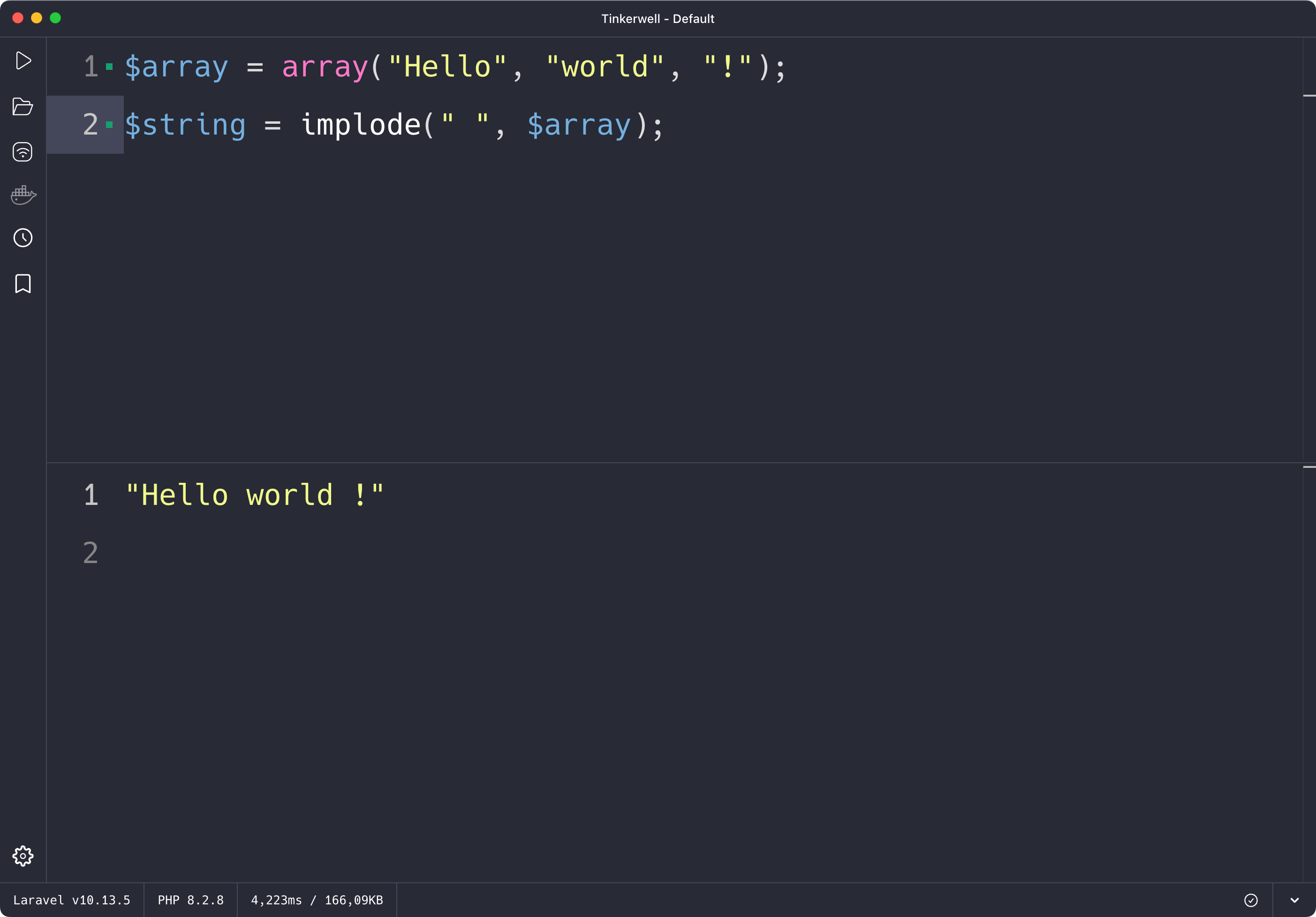Tinkerwell
Go back to Blog

# How to use implode() in PHP (with examples)

The implode() function in PHP is used to join together an array of strings into a single string. This function takes two arguments: the first is the glue, which is the string that will be placed between each array element, and the second is the array of strings that you want to join together. The resulting string will be returned by the function.

The easiest way to play around with PHP functions or run code within the context of your application or website is by using Tinkerwell – the code runner for PHP.

Here is an example of how you might use the implode() function in PHP:

``\$array = array("Hello", "world", "!");\$string = implode(" ", \$array); // \$string will be "Hello world !"``In the example above, we have an array of strings, and we use the implode() function to join them together into a single string, with a space character as the glue. The resulting string is stored in the \$string variable.

Another way to use the implode() function is to pass in an empty string as the glue:

``\$array = array("Hello", "world", "!");\$string = implode("", \$array); // \$string will be "Helloworld!"``

In this example, we have the same array of strings, but we are using an empty string as the glue. This causes the implode() function to concatenate the array elements without any separator characters between them.

You can also use the implode() function to convert an array of numbers into a string. For example:

``\$array = array(1, 2, 3, 4, 5);\$string = implode("-", \$array); // \$string will be "1-2-3-4-5"``

In the example above, we have an array of numbers, and we use the implode() function to convert it into a string. The resulting string is a list of numbers separated by hyphens.

You can also use the implode() function to convert an array of booleans into a string. For example:

``\$array = array(true, false, true, false);\$string = implode(" ", \$array); // \$string will be "1 0 1 0"``

In this example, we have an array of booleans, and we use the implode() function to convert them into a string. The resulting string is a list of "1" and "0" values, with a space character between each value.

In conclusion, the implode() function in PHP is a useful tool for joining together an array of strings into a single string. It takes two arguments: the glue, which is the string that will be placed between each array element, and the array of strings that you want to join together. The resulting string is returned by the function.

## Tinkerwell: The code runner for PHP

The must-have companion to your favorite IDE. Quickly iterate on PHP code within the context of your web application.